Custom SearchDEPENDENT EVENTS In some cases one event is dependent on another; that is, two or more events are said to be dependent if the occurrence or nonoccurrence of one of the events affects the probabilities of occurrence of any of the others. Consider that two or more events are dependent. If p1 is the probability of a first event; p2 the probability that after the first happens, the second will occur; p3 the probability that after the first and second have happened, the third will occur; etc., then the probability that all events will happen in the given order is the product p1 - p2 - p3. EXAMPLE: A box contains 3 white marbles and 4 black marbles. What is the probability of drawing 2 black marbles and 1 white marble in succession without replacement? SOLUTION: On the first draw the probability of drawing a black marble is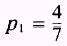On the second draw the probability of drawing a black marble is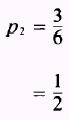On the third draw the probability of drawing a white marble is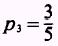Therefore, the probability of drawing 2 black marbles and 1 white marble is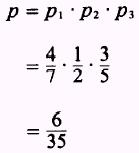EXAMPLE: Slips numbered 1 through 9 are placed in a box. If 2 slips are drawn, without replacement, what is the probability that 1. both are odd? 2. both are even? SOLUTION: 1. The probability that the first is odd is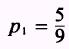and the probability that the second is odd isTherefore, the probability that both are odd is2. The probability that the first is even is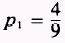and the probability that the second is even is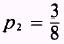Therefore, the probability that both are even isA second method of solution involves the use of combinations. 1. A total of 9 slips are taken 2 at a time and 5 odd slips are taken 2 at a time; therefore,2. A total of 9C2 choices and 4 even slips are taken 2 at a time; therefore,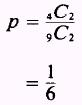PRACTICE PROBLEMS: In the following problems assume that no replacement is made after each selection: 1. A box contains 5 white and 6 red marbles. What is the probability of successfully drawing, in order, a red marble and then a white marble? 2. A bag contains 3 red, 2 white, and 6 blue marbles. What is the probability of drawing, in order, 2 red, 1 blue, and 2 white marbles? 3. Fifteen airmen are in the line crew. They must take care of the coffee mess and line shack cleanup. They put slips numbered 1 through 15 in a hat and decide that anyone who draws a number divisible by 5 will be assigned the coffee mess and anyone who draws a number divisible by 4 will be assigned cleanup. The first person draws a 4, the second a 3, and the third an 11. What is the probability that the fourth person to draw will be assigned a. the coffee mess? b. the cleanup? ANSWERS: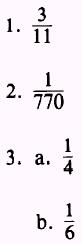Integrated Publishing, Inc. - A (SDVOSB) Service Disabled Veteran Owned Small Business